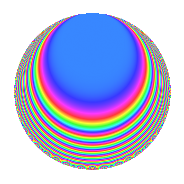# Properties

 Label 800.1.boLevel 800 Weight 1 Character orbit bo Rep. character $$\chi_{800}(33,\cdot)$$ Character field $$\Q(\zeta_{20})$$ Dimension 8 Newforms 1 Sturm bound 120 Trace bound 0

# Related objects

## Defining parameters

 Level: $$N$$ = $$800 = 2^{5} \cdot 5^{2}$$ Weight: $$k$$ = $$1$$ Character orbit: $$[\chi]$$ = 800.bo (of order $$20$$ and degree $$8$$) Character conductor: $$\operatorname{cond}(\chi)$$ = $$25$$ Character field: $$\Q(\zeta_{20})$$ Newforms: $$1$$ Sturm bound: $$120$$ Trace bound: $$0$$

## Dimensions

The following table gives the dimensions of various subspaces of $$M_{1}(800, [\chi])$$.

Total New Old
Modular forms 72 8 64
Cusp forms 8 8 0
Eisenstein series 64 0 64

The following table gives the dimensions of subspaces with specified projective image type.

$$D_n$$ $$A_4$$ $$S_4$$ $$A_5$$
Dimension 8 0 0 0

## Trace form

 $$8q + O(q^{10})$$ $$8q + 2q^{13} + 2q^{17} + 2q^{25} - 2q^{37} - 2q^{45} - 2q^{53} - 2q^{65} - 2q^{73} + 2q^{81} - 8q^{85} - 10q^{89} - 2q^{97} + O(q^{100})$$

## Decomposition of $$S_{1}^{\mathrm{new}}(800, [\chi])$$ into irreducible Hecke orbits

Label Dim. $$A$$ Field Image CM RM Traces $q$-expansion
$$a_2$$ $$a_3$$ $$a_5$$ $$a_7$$
800.1.bo.a $$8$$ $$0.399$$ $$\Q(\zeta_{20})$$ $$D_{20}$$ $$\Q(\sqrt{-1})$$ None $$0$$ $$0$$ $$0$$ $$0$$ $$q+\zeta_{20}^{7}q^{5}+\zeta_{20}q^{9}+(-\zeta_{20}^{8}+\zeta_{20}^{9}+\cdots)q^{13}+\cdots$$